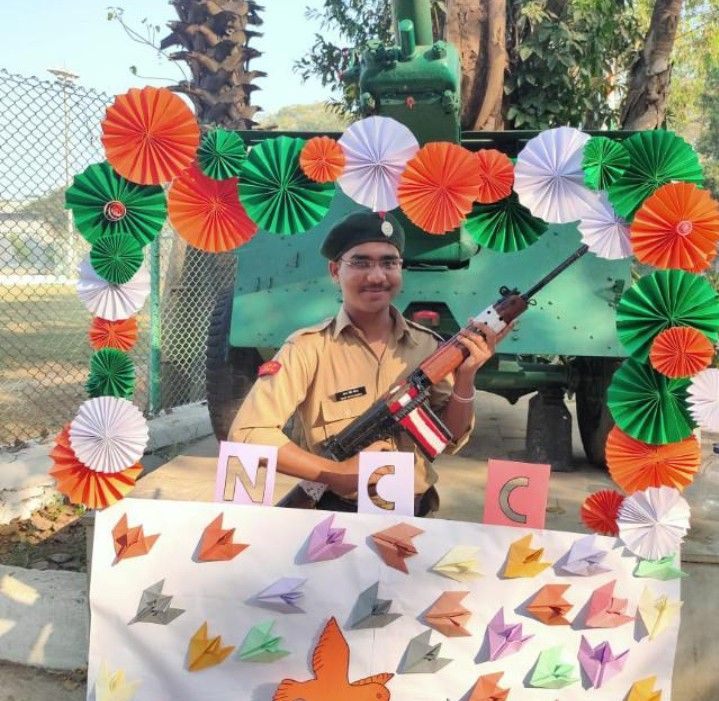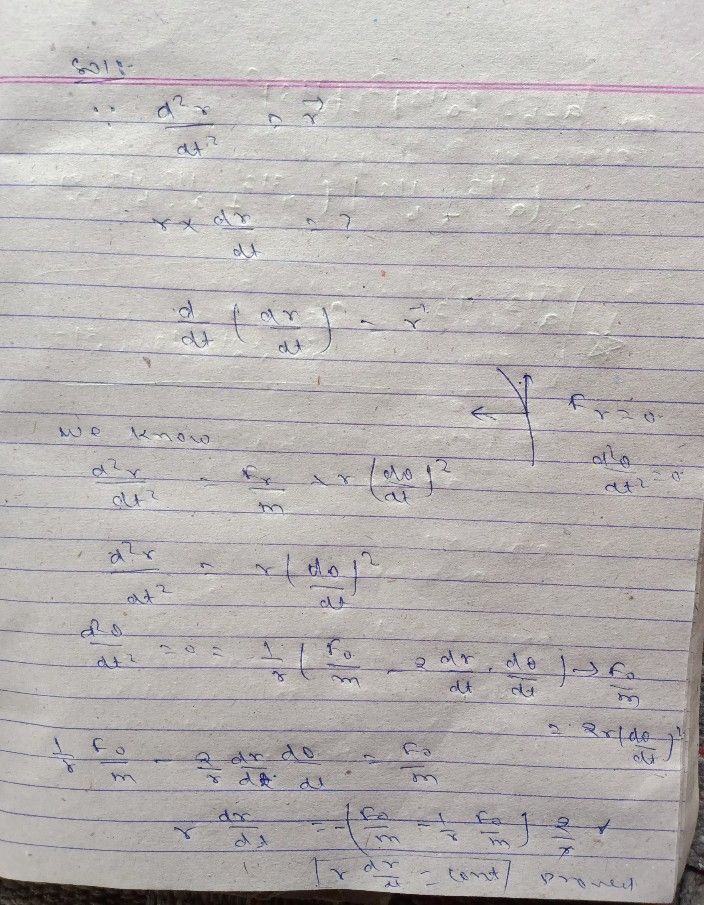Symbol
Problem$1$ $1t$ $1f\dfrac {d^{2}r} {dt^{2}}=t,$ then show that $r\times \dfrac {d\pi } {dt}$ is a constant vector.
Other
Search count: 106
SolutionQanda teacher - udaysinghclear sir
all the bestStudent
This is a math's question in my book.... you apply physics in it.... is this the one and only process??Qanda teacher - udaysingh
yes its math no phy enginering mathamatics
in this question also use some concept phy dear
than finally provedStudent
Ok... thanks for your contribution... ❤️Qanda teacher - udaysingh
blcm sir
plz evalute ansStudent
From where??